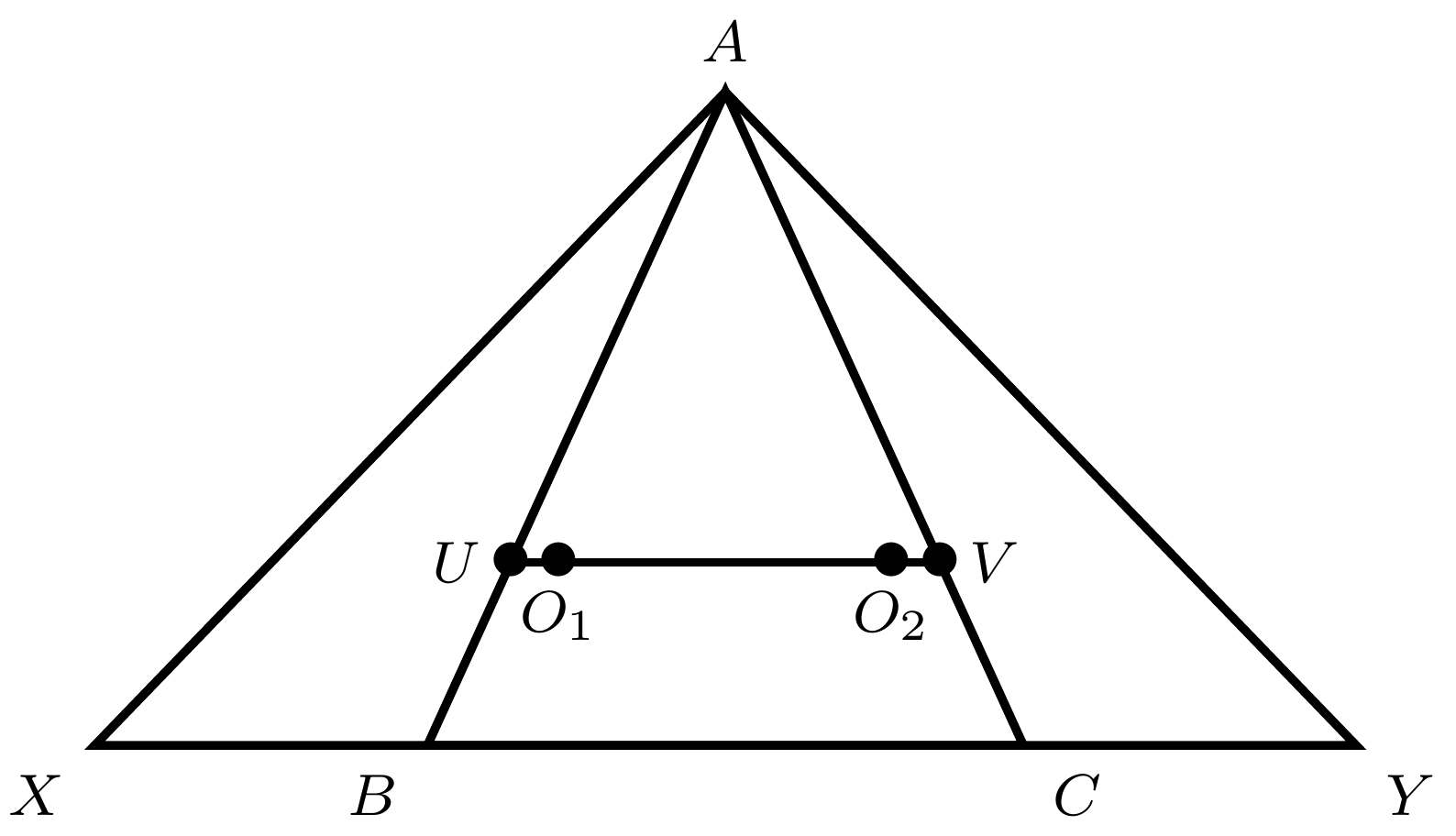PowerOfPointTheorem Difficult
2016

Problem - 3301

As shown, points $X$ and $Y$ are on the extension of $BC$ in $\triangle{ABC}$ such that the order of these four points are $X$, $B$, $C$, and $Y$. Meanwhile, they satisfy the relation $BX\cdot AC = CY\cdot AB$. Let $O_1$ and $O_2$ be the circumcenters of $\triangle{ACX}$ and $\triangle{ABY}$, respectively. If $O_1O_2$ intersects $AB$ and $AC$ at $U$ and $V$, respectively, show that $\triangle{AUV}$ is isosceles.The solution for this problem is available for $0.99. You can also purchase a pass for all available solutions for$99.